Maths
Study Material

# Inverse of a matrix using elementary row operations

Many a times, we need to find inverse of a given matrix. Let us look at one of the easiest ways to find the inverse of an invertible matrix.

6 minutes long
Posted by Tanwir Silar, 4/8/2021Hesap Oluştur

Got stuck on homework? Get your step-by-step solutions from real tutors in minutes! 24/7. Unlimited.

The need to find the inverse of a matrix in matrix algebra is immense. This brings us to one of the easiest ways to find the inverse of a matrix – using elementary row operations.

## What are elementary operations of a matrix?

1. Interchange of any two rows or two columns. Symbolically written as,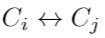and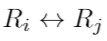2. The multiplication of the elements of any row or column by a non-zero number. Symbolically shown as,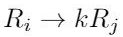and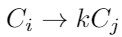3. The addition to the elements of any row or column, the corresponding elements of any other row or column multiplied by any non zero number. Represented as,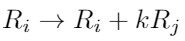and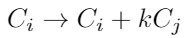## Invertible Matrices

A square matrix A is said to invertible if its determinant has a non-zero value, i.e., A is non-singular.
For a square matrix A of order n, its inverse is denoted by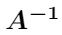and has an order n. The product of a matrix and its inverse results to the Identity matrix of order n.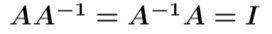We can observe that, if B is the inverse of A, then A is the inverse of B.
The notion of inverse can be used to calculate an unknown matrix within multiplication.

Example:
To know the values of X, we need to multiply both sides of the equation with the inverse of A. Here, the order of X, A, B is same.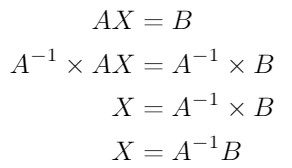If A and B are known, then X can be calculated using above steps.

## Elementary row operations – Inverse of matrix

Before diving into calculating the inverse of a matrix, let us know more about what operations should be applied to the product AB, if elementary operations (transformations) are performed on X, so that the equation X = AB holds. Row operations carried out on X should also be performed on the first matrix, A, of the product AB. Similarly, if column operations were carried out on X then they should also be carried on the second matrix, B, of the product B.

Procedure
1. We write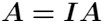, and then apply a sequence of row operations on A till we get,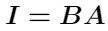.

Example:
Using elementary operations, calculate the inverse of the matrix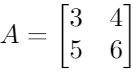Solution:
We start by writing.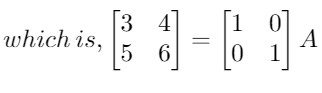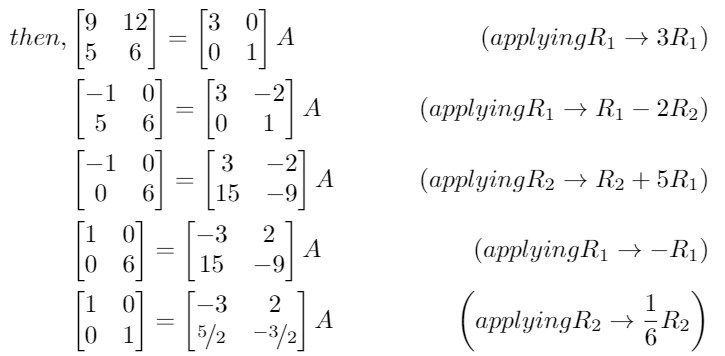We have been able to writeaswhere B is the inverse of matrix A.
Hence, the inverse is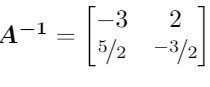We can validate the answer by multiplying it with A, as.